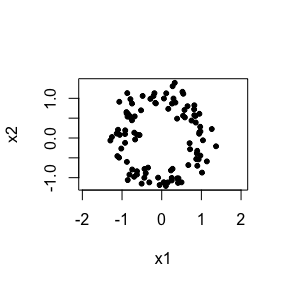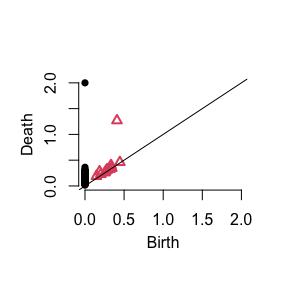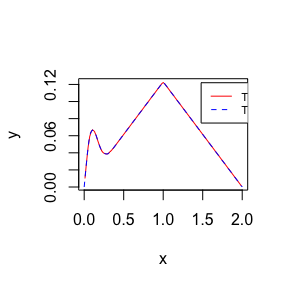# TDAvec vignette

The TDAvec package provides implementations of several vector summaries of persistence diagrams studied in Topological Data Analysis (TDA). Each is obtained by discretizing the associated summary function computed from a persistence diagram. The summary functions included in this package are

1. Persistence landscape function
2. Persistence silhouette function
3. Persistent entropy summary function
4. Euler characteristic curve
5. Betti curve
6. Normalized life curve
7. Persistence surface
8. Persistence block

For improved computational efficiency, all code behind the vector summaries is written in C++ using the Rcpp package. Whenever applicable, when compare our code with existing implementations in terms of accuracy and run-time cost. In this vignette, we illustrate the basic usage of the TDAvec package using simple examples.

Let’s first load the required libraries.

library(TDAvec)
library(TDA) # to compute persistence diagrams
library(microbenchmark) # to compare computational costs

Now, we generate a 2D point cloud of size 100 sampled uniformly from a unit circle with added Gaussian noise:

N <- 100 # point cloud size
set.seed(123)
X <- circleUnif(N) + rnorm(2*N,mean = 0,sd = 0.2)
# plot the point cloud
plot(X,pch = 20,asp = 1)Next, we use the TDA package to compute the persistence diagram (PD) using the Vietoris-Rips filtration built on top of the point cloud $$X$$.

D <- ripsDiag(X,maxdimension = 1,maxscale = 2)$diagram sum(D[,1]==0) # number of connected components #>  100 sum(D[,1]==1) # number of loops #>  13 sum(D[,1]==2) # number of voids #>  0 In the ripsDiag() function, maxdimension is the maximum homological dimension of the topological features to be computed (connected components if maxdimension=0; connected components and loops if 1; connected components, loops and voids if 2, etc.) maxscale is the maximum value of the scale parameter of the filtration (which we set equal to 2 since the points are sampled from a circle with diameter 2). The persistence diagram $$D$$ has 100 connected components (the same as the point cloud size), 13 loops (one with long life-span, the rest are short-lived) and 0 voids along with their birth and death values. To plot the diagram, we can use the plot() function. plot(D)In the plot, the solid dots and red triangles represent connected components and loops respectively. Let’s compute a vector summary of the persistence landscape (PL) for homological dimension $$H_0$$. # sequence of scale values to vectorize the summary function scaleSeq = seq(0,2,length.out=11) # compute the PL vector summary for homological dimension H_0 computePL(D,homDim = 0,scaleSeq,k=1) #>  0.0 0.2 0.4 0.6 0.8 1.0 0.8 0.6 0.4 0.2 0.0 Here, the vectorization is performed by evaluating the PL function at each element of scaleSeq (i.e. $$0.0,0.2,0.4,\ldots,2.0$$) and arranging the values into a vector. The parameter k in computePL() is the order of the persistence landscape function (by default $$k=1$$). To compute an $$H_1$$ vector summary, set the homDim argument equal to 1: # compute the PL vectory summary for homological dimension H_1 computePL(D,homDim = 1,scaleSeq,k=1) #>  0.000000000 0.014217630 0.000931983 0.191114536 0.391114536 0.269212150 #>  0.069212150 0.000000000 0.000000000 0.000000000 0.000000000 The TDA package also provides an implementation of the persistence landscapes. Below we compare the two implementations in terms of accuracy of results and run-time cost. pl1 <- computePL(D,homDim = 0,k=1,scaleSeq) pl2 <- as.vector(landscape(D,dimension = 0,KK = 1, tseq = scaleSeq)) all.equal(pl1,pl2) # -> TRUE (the results are the same) #>  TRUE compCost <- microbenchmark( computePL(D,homDim = 0,k=1,scaleSeq), landscape(D,dimension = 0,KK = 1, tseq = scaleSeq), times = 500 ) sm <- summary(compCost) costRatioPL <- sm$mean/sm$mean # ratio of computational time means For homological dimension $$H_0$$, it took TDA::landscape() about 228 times more time than TDAvec::computePL(). To discretize all the other univariate summary functions (i.e., persistence silhouette, persistent entropy summary function, Euler characteristic curve, normalized life curve and Betti curve), we employ a different vectorization scheme. Instead of evaluating a summary function at increasing scales and arranging the values into a vector, we compute the average values of the summary function between two consecutive scale points using integration. More specifically, if $$f$$ is a (univariate) summary function and $$t_1,t_2,\ldots,t_n$$ are increasing scale values, we discretize $$f$$ as: $\begin{equation} \Big(\frac{1}{\Delta t_1}\int_{t_1}^{t_2}f(t)dt,\frac{1}{\Delta t_2}\int_{t_2}^{t_3}f(t)dt,\ldots,\frac{1}{\Delta t_{n-1}}\int_{t_{n-1}}^{t_n}f(t)dt\Big)\in\mathbb{R}^{n-1}, \end{equation}$ where $$\Delta t_k=t_{k+1}-t_k$$, $$k=1,\ldots,n-1$$. For the above five summary functions, the computation of integrals can be done analytically and efficiently implemented. Note that in this case, vector summaries lie in $$\mathbb{R}^{n-1}$$, where the dimension is one less than the number of scale values. The TDA::silhouette() function vectorizes the persistence silhouette function the same way as the TDA::landscape() function does. However, TDA::silhouette() and TDAvec::computePS() provide similar results if a dense grid of scale values is used for vectorization: n <- 101 scaleSeq = seq(0,2,length.out=n) ps1 <- computePS(D,homDim = 0, p = 1,scaleSeq) ps2 <- as.vector(silhouette(D,dimension = 0,p = 1,tseq = scaleSeq)) # plot two vector summaries plot(scaleSeq[1:(n-1)]+1/(n-1),ps1, type="l",col="red",xlab="x",ylab="y",lty=1) lines(scaleSeq,ps2,type='l',col='blue',lty=2) legend(1.48, 0.122, legend=c("TDAvec","TDA"), col=c("red","blue"),lty=1:2,cex=0.7)compCost <- microbenchmark( computePS(D,homDim = 0,p = 1,scaleSeq), silhouette(D,dimension = 0,p = 1,tseq = scaleSeq), times = 500 ) sm <- summary(compCost) costRatioPS <- sm$mean/sm\$mean
print(costRatioPS)
#>  3.554547

The $$p$$ in TDAvec::computePS()and TDA::silhouette() is the power of the weights for the silhouette function (by default $$p=1$$). For the above example, the former was about 4 times faster to than the latter for homological dimension $$H_0$$.

The syntax and usage of the remaining univariate summary functions are very similar.

# sequence of scale values to vectorize the summary function
scaleSeq = seq(0,2,length.out=11)

# Persistent Entropy Summary (PES) function
# compute PES for homological dimension H0
computePES(D,homDim = 0,scaleSeq)
#>   4.8268301 1.0173158 0.3720045 0.3720045 0.3720045 0.3720045 0.3720045
#>   0.3720045 0.3720045 0.3720045
# compute PES for homological dimension H1
computePES(D,homDim = 1,scaleSeq)
#>   0.05677674 0.26458225 0.33845861 0.34789260 0.34789260 0.34789260
#>   0.12039198 0.00000000 0.00000000 0.00000000

# Euler Characteristic Curve (ECC)
computeECC(D,maxhomDim = 1,scaleSeq) # maxhomDim = maximal homological dimension considered
#>   65.64393340  5.93893485 -0.02801848  0.00000000  0.00000000  0.00000000
#>    0.65393925  1.00000000  1.00000000  1.00000000

# Vector of Averaged Bettis (VAB) - a vectorization of Betti Curve
# compute VAB for homological dimension H0
computeVAB(D,homDim = 0,scaleSeq)
#>   65.928137  7.313179  1.000000  1.000000  1.000000  1.000000  1.000000
#>    1.000000  1.000000  1.000000
# compute VAB for homological dimension H1
computeVAB(D,homDim = 1,scaleSeq)
#>   0.2842034 1.3742440 1.0280185 1.0000000 1.0000000 1.0000000 0.3460608
#>   0.0000000 0.0000000 0.0000000

# Normalized Life (NL) Curve
# compute NL for homological dimension H0
computeNL(D,homDim = 0,scaleSeq)
#>   0.8171309 0.2341008 0.1230901 0.1230901 0.1230901 0.1230901 0.1230901
#>   0.1230901 0.1230901 0.1230901
# compute NL for homological dimension H1
computeNL(D,homDim = 1,scaleSeq)
#>   0.01309940 0.06318557 0.68241758 0.71307326 0.71307326 0.71307326
#>   0.24676667 0.00000000 0.00000000 0.00000000

To discretize the persistence surface and persistence block, we first need to switch from the birth-death to the birth-persistence coordinates.

D[,3] <- D[,3] - D[,2]
colnames(D) <- "Persistence"

The resulting vector summaries are called the persistence image (PI) and the vectorized of persistence block (VPB) respectively.

# Persistence Image (PI)
resB <- 5 # resolution (or grid size) along the birth axis
resP <- 5 # resolution (or grid size) along the persistence axis
# find min and max persistence values
minPH0 <- min(D[D[,1]==0,3]); maxPH0 <- max(D[D[,1]==0,3])
# construct one-dimensional grid of scale values
ySeqH0 <- seq(minPH0,maxPH0,length.out=resP+1)
# default way of selecting the standard deviation sigma of the Gaussians on top of each point of the diagram
sigma <- 0.5*(maxPH0-minPH0)/resP
# compute PI for homological dimension H_0
computePI(D,homDim=0,xSeq=NA,ySeqH0,sigma)
#>  4.56670703 0.96562735 0.01135007 0.02272340 0.47724987

# Vectorized Persistence Block (VPB)
tau <- 0.3 # parameter in [0,1] which controls the size of blocks around each point of the diagram
# compute VPB for homological dimension H_0
computeVPB(D,homDim = 0,xSeq=NA,ySeqH0,tau)
#>  3.90196609 0.06216766 0.00000000 0.77425106 1.80204108

Since the $$H_0$$ features all have the birth value of zero in this case, a one-dimensional grid of scale values is used for vectorization.

For homological dimension $$H_1$$, the birth values are not all the same and therefore the vectorization is performed over a two-dimensional grid. For the VPB summary, since the blocks around each point of the persistence diagram have different sizes, we construct the grid with scale values spread out non-uniformly (i.e. the rectangular grid cells have different dimensions). In experiments, this construction of the grid tends to provide better performance over the grid with equal cell sizes.

# PI
# find min & max birth and persistence values
minBH1 <- min(D[D[,1]==1,2]); maxBH1 <- max(D[D[,1]==1,2])
minPH1 <- min(D[D[,1]==1,3]); maxPH1 <- max(D[D[,1]==1,3])
xSeqH1 <- seq(minBH1,maxBH1,length.out=resB+1)
ySeqH1 <- seq(minPH1,maxPH1,length.out=resP+1)
sigma <- 0.5*(maxPH1-minPH1)/resP
# compute PI for homological dimension H_1
computePI(D,homDim=1,xSeqH1,ySeqH1,sigma)
#>   4.371601e-02 4.955567e-02 4.512378e-02 3.299957e-02 1.864442e-02
#>   7.527654e-03 7.760847e-03 6.305986e-03 4.250972e-03 2.278547e-03
#>  6.038006e-05 5.841014e-05 4.427399e-05 3.094726e-05 2.021064e-05
#>  1.834675e-04 8.921009e-04 2.714215e-03 5.171823e-03 6.175378e-03
#>  3.853840e-03 1.874023e-02 5.701774e-02 1.086451e-01 1.297270e-01

# VPB
xSeqH1 <- unique(quantile(D[D[,1]==1,2],probs = seq(0,1,by=0.2)))
ySeqH1 <- unique(quantile(D[D[,1]==1,3],probs = seq(0,1,by=0.2)))
tau <- 0.3
# compute VPB for homological dimension H_1
computeVPB(D,homDim = 1,xSeqH1,ySeqH1,tau)
#>   0.0000000000 0.0004995598 0.0090337733 0.0305098818 0.0057973687
#>   0.0095194496 0.0261053157 0.0203673036 0.0042870130 0.0107446149
#>  0.0425768331 0.1053468321 0.1458807241 0.0000000000 0.0000000000
#>  0.0328035481 0.0276785592 0.1089088594 0.1318822254 0.0168304895
#>  0.2939394561 0.3162986126 0.3344510989 0.3567486932 0.3636226941

As a note, the code for computePI() is adopted from the pers.image() function (available in the kernelTDA package) with minor modifications. For example, pers.image() uses a one-dimensional grid for homological dimension $$H_0$$ regardless of the filtration type. In contrast, computePI() uses a one-dimensional grid only if additionally the birth values are the same (which may not be true for some filtrations such as the lower-star filtration). Moreover, pers.image() uses a square grid (e.g., 10 by 10) for vectorization, whereas computePI() is not limited to such a grid and can compute vector summaries using a rectangular grid (e.g., 10 by 20).